Energy Management of Hybrid Power System PV Wind and Battery Based Three Level Converter

Energy Management of Hybrid Power System PV Wind and Battery Based Three Level Converter

Mostefa KoulaliMohamed Mankour Karim Negadi Abdelkader Mezouar

University Tahar Moulay of Saida, Adresse: BP 138 cité ENNASR 20000, Saida, Algeria

University Ibn Khaldoun of Tiaret BP 78 Tiaret 14000, Faculty of Applied Sciences, Laboratory of Energy and Computer Engineering L2GEGI, Tiaret, Algeria

Corresponding Author Email:
mostefa.koulali@univ-tiaret.dz
Page:
297-304
|
DOI:
https://doi.org/10.18280/ti-ijes.632-426
14 February 2019
|
Accepted:
5 May 2019
|
Published:
30 June 2019
| Citation

OPEN ACCESS

Abstract:

This paper presents a study of the hybrid system consisting a three energy sources, namely wind energy, photovoltaic power source PV and Battery. Each of the three energy sources is controlled so as to deliver energy at optimum efficiency. A multilevel inverter was used in the system to improving the quality of energy injected into the AC load. Fuzzy logic control is used to achieve maximum power to a DC bus voltage. A management system is designed to manage the power flow between the system components in order to satisfy the load requirements. The considered system was implemented in the Matlab/Simulink, the results show the effectiveness of the proposed method and can be realized with experimented setup.

Keywords:

wind energy, photovoltaic PV, battery, hybrid system, MPPT tracking, three level inverter, fuzzy logic control (FLC)

1. Introduction

Renewable energy sources have received greater attention during the past few decades and considerable efforts have been made to develop efficient renewable energy conversion system. The major objectives of these approaches are to have reduced environmental damage, conservation of energy, exhaustible sources and increased safety. The renewable energy systems can be used to supply power either directly to a utility grid or to an isolated load. The stand-alone systems find wider applications in isolated areas which are far away from the utility grid .

The integration of Energy storage systems (ESSs) have some significant applications in operations like grid stabilization, stable power quality, load shifting, grid operational support and smooth power injection to grid. Several power smoothing methods have been introduced in literature , battery energy storage system (BESS) is selected as energy storage and incorporated into wind, PV to maintain power and energy balance as well as to improve power quality.

In our research, the proposed Energy Management of Hybrid Power System PV/Wind and Battery Based Three Level Converter consists of a wind energy conversion system (WECS)/PV generator as the primary energy source and a battery energy storage system (as short and medium, time storage devices).

They are all connected to a DC voltage link, and a three phase three level Neutral Point Clamping (NPC) converter is used to connect the whole system to the load. The DC coupled structure makes the overall system more flexible since the number and the types of energy sources can be freely chosen and it requires fewer controllers as no synchronization is needed to integrate the energy sources.

For the control of the battery using the information compared between the reference DC voltage connection and the measured DC voltage, a reference battery current

is obtained, the battery current is controlled by hysteresis in order to control the bidirectional converter duty cycle S1 and S2 taking into account the state of charge of the SOC battery.

Regarding the control of the three-level inverter connected to the load, we used fuzzy logic control (FLC) to ensure the RMS value of the load voltage.

The structure of the presented work is organized as follow: The description of the proposed hybrid system approach and the physical modeling different part of our system with their equations model is set in section 2. The control of different component is set in section 3. The simulations results of the studied are presented in section 4. Section 5 summarizes the work done in the conclusion.

2. Description of Proposed Hybrid Power System

The Figure 1 describes the hybrid scheme of solar-wind and battery with the proposed fuzzy logic controller.

1.png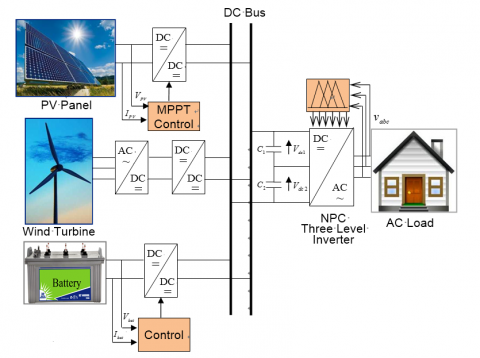Figure 1. Proposed configuration of hybrid system with battery

2.1 Modeling of solar PV

PV system is based on solar energy, where PV cell is the most basic generation part in PV. As Figure 2 shows, the PV cell is formed from a diode and a current source was connected antiparallel with a series resistance .

The relation of the current and voltage in the single-diode cell can be written as follows:

${{I}_{PV}}={{I}_{ph}}-{{I}_{0}}\left( \exp \left( \frac{q\left( {{V}_{PV}}+{{R}_{s\bmod }}{{I}_{PV}} \right)}{AKT} \right)-1 \right)$(1)

2.pngFigure 2. Equivalent circuit of PV cell

2.2 DC/DC boost converter and maximum power point tracking (MPPT)

A boost converter is a step up DC/DC converter which increases the solar voltage to a desired output voltage as required by load. The configuration is shown in Figure 3, which consists of a DC input voltage

, inductor L, switch S, diode D1, capacitor C for filter, and load resistance R.

When the switch S is ON the boost inductor stores the energy fed from the input voltage source and during this time the load current is maintain by the charged capacitor so that the load current should be continuous. When the switch S is OFF the input voltage and the stored inductor voltage will appear across the load hence the load voltage is increased. Hence, the load voltage is depends upon weather switch S in ON or OFF and this is depends upon the duty ratio D.

3.png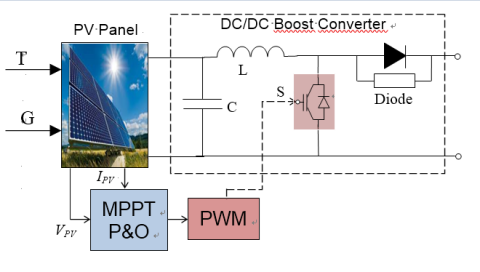Figure 3. Boost converter with MPPT control

The solar panel efficiency is increased by the use MPPT technique. The MPPT is the application of maximum power transfer theorem which says that the load will receive maximum power when the source impedance is equal to load impedance. The MPPT is a device that extracts maximum power from the solar cell and changes the duty ratio of DC/DC converter in order to match the load impedance to the source.

2.3 Wind energy system

(1) Wind turbine

The wind generator consists of a wing which captures the kinetic energy of the wind coupled directly with a synchronous generator which delivers on a DC bus via a diode rectifier; this is the retained structure for this modeling and simulation work.

The mechanical power of a wind turbine is expressed as follows [4-5]:

${{P}_{mech}}=\frac{1}{2}\rho {{A}_{t}}{{C}_{P}}V_{\omega }^{3}$   (2)

The power coefficient is:

${{C}_{p}}=\frac{2{{P}_{wind}}}{\lambda SV_{wind}^{3}}$  (3)

${{T}_{wind}}={{T}_{mec}}=\frac{1}{2}\frac{{{C}_{P}}(\lambda )\rho RSV_{wind}^{2}}{\lambda }$  (4)

(2) PMSG model

The permanent magnet synchronous machine model used is modeled by the following equations :

$\left[ \begin{matrix} {{V}_{d}} \\ {{V}_{q}} \\\end{matrix} \right]=\left[ \begin{matrix} {{R}_{c}} & -\omega {{L}_{c}} \\ \omega {{L}_{c}} & {{R}_{c}} \\\end{matrix} \right]\left[ \begin{matrix} {{i}_{d}} \\ {{i}_{q}} \\\end{matrix} \right]+{{L}_{c}}\frac{d}{dt}\left[ \begin{matrix} {{i}_{d}} \\ {{i}_{q}} \\\end{matrix} \right]+\left[ \begin{matrix} {{e}_{d}} \\ {{e}_{q}} \\\end{matrix} \right]$    (5)

The electromagnetic torque is given by:

${{T}_{em}}=\frac{p}{\omega }\left( {{i}_{q}}{{e}_{q}} \right)=p{{\psi }_{f}}{{i}_{q}}$  (6)

The mechanical equation for the PMSG is expressed as:

$\frac{Jd{{\omega }_{r}}}{dt}={{T}_{em}}-{{T}_{l}}-f{{\omega }_{r}}$   (7)

3. Control of System Components

The hybrid system shown in Figure 1 contains control strategies that will be developed in this section.

3.1 Modeling and control of the battery

(1) Battery modeling

Several authors have proposed models for the battery and the results of experiments carried out on lead/acid batteries deduce a model named “CIEMAT model” representing the battery operation during the charge, discharge and overcharge processes. In our case study, from the carried out experiments, a validated model is proposed with respect to the battery capacity for any size and type of Nickel Metal Hydride battery .

This model represented by an equivalent circuit model contains a voltage source which is the open circuit voltage V, in series with an internal resistance R. Thus, the output voltage of the battery is:

${{V}_{bat}}=V-R{{I}_{bat}}$  (8)

where, the both Vbat  and  Ibat  depend on the battery state of charge (SOC), temperature and internal resistance variations R .

4.png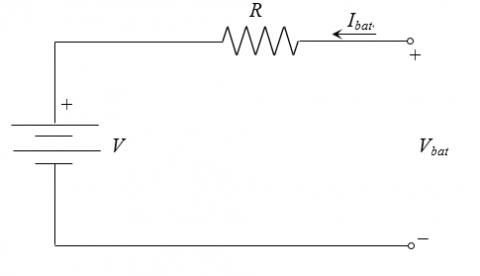Figure 4. The battery controls

In this study, this simple model based on the CIEMAT model for the battery is considered as enough accurate to assess power management objectives and to compare performance of several strategies. During the charging and discharging process, the state of charge (SOC) in terms of time (t) can be described by 

$SOC\left( t \right)=\left\{ \begin{matrix} SOC\left( t-\Delta t \right)+{{P}_{bat}}.\frac{{{\eta }_{ch}}}{{{C}_{n}}.{{V}_{dc}}}.\text{ }\!\!\Delta\!\!\text{ }t \\ SOC\left( t-\Delta t \right)+{{P}_{bat}}.\frac{1}{{{\eta }_{dis}}.{{C}_{n}}.{{V}_{dc}}}.\Delta t \\\end{matrix} \right.$    (9)

where, Δt is the time step,  Pbat  represents the battery power,  Cn  is the nominal capacity of the battery,  ${{\eta }_{ch}}$ and ${{\eta }_{dis}}$  are respectively the battery efficiencies during charging and discharging phase.  Vdc  denotes the nominal DC bus voltage. At any time, step Δt, the SOC must comply with the following constraints

$~SO{{C}_{\min }}\le SOC\left( t \right)\le SO{{C}_{\max }}$    (10)

where, SOCmin and SOCmax are maximum and minimum allowable storage capacities, respectively.

(2) Battery control

5.pngFigure 5. The battery controls

The objective of the control system is to regulate the battery current in order to obtain the required power. Charging and discharging current limits and maximum SOC limitations are also included in the model. The BESS is connected to the DC grid via a bi-directional Buck-Boost DC/DC converter, as shown in Figure 5.

The BESS will operate in charging, discharging or floating modes depending on the energy requirements and these modes are managed according to the DC bus voltage at the BESS point of coupling. Consequently, the BESS is required to provide necessary DC voltage level under different operating modes of the microgrid. When charging, switch S2 is activated and the converter works as a boost circuit; otherwise, when discharging, switch S1 is activated and the converter works as a buck circuit. When the voltage at the DC link is lower than the voltage reference, switch S1 is activated. Alternatively, when the voltage at the DC link is higher than the voltage reference, switch S2 is activated. The PV-battery system response to transient variations is characterized by an inherent time constant. In such cases, capacitors along the DC grid can act as virtual inertia to supply the shortfall or absorb the surplus of energy [9-11].

The DC-link power balance can be expressed by the following differential equation:

${{V}_{dc}}{{i}_{dc}}={{P}_{PV}}+{{P}_{W}}+{{P}_{bat}}-{{P}_{load}}$    (11)

Neglecting the losses in the power converters, battery, filtering inductors and transformer and also the harmonics due to switching actions, the power balance of the integrated hybrid distributed generation system (DGS) with energy storage is governed by:

${{V}_{dc}}{{i}_{dc}}=C{{V}_{dc}}\frac{d{{V}_{dc}}}{dt}={{P}_{PV}}+{{P}_{W}}+{{P}_{bat}}-{{P}_{load}}$   (12)

The objective of the battery converter is to maintain constant voltage at the DC link, so the ripple in the capacitor voltage is much lower than the steady-state voltage.

If the powers injected by the two back-to-back voltage source converters (VSC) are assumed constant at any particular instant, the power from the battery is responsible for adjusting the capacitor voltage.

3.2 Three-level inverter modeling

6.pngFigure 6. Three-level inverter

A three-level inverter differs from a conventional two-level inverter in that it is capable of producing three different levels of output phase voltage. The structure of a three-level neutral point clamped inverter is shown in Figure 6. When switches 1 and 2 are on the output is connected to the positive supply rail. When switches 3 and 4 are on, the output is connected to the negative supply rail. When switches 2 and 3 are on, the output is connected to the supply neutral point via one of the two clamping diodes [12, 13].

The functions  $F_{km}^{b}$  of connection are given by:

$\left\{ \begin{matrix} F_{k1}^{b}={{F}_{k1}}.{{F}_{k2}} \\ F_{k0}^{b}={{F}_{k3}}.{{F}_{k4}} \\\end{matrix} \right.$    (13)

where,  m=1:  the upper half arm and m=0: the lower half arm.

The phase voltage  ${{V}_{AO}},{{V}_{BO}},{{V}_{CO}}$  can be written as:

$\left\{ \begin{matrix} {{V}_{AO}}=F_{11}^{b}{{V}_{c1}}-F_{10}^{b}{{V}_{c2}} \\ {{V}_{BO}}=F_{21}^{b}{{V}_{c1}}-F_{20}^{b}{{V}_{c2}} \\ {{V}_{CO}}=F_{31}^{b}{{V}_{c1}}-F_{30}^{b}{{V}_{c2}} \\\end{matrix} \right.$    (14)

Simple output voltages are written as:

$\left[ \begin{matrix} {{V}_{A}} \\ {{V}_{B}} \\ {{V}_{C}} \\\end{matrix} \right]=\frac{1}{3}\left[ \begin{matrix} 2 & -1 & -1 \\ -1 & 2 & -1 \\ -1 & -1 & 2 \\\end{matrix} \right]\left\{ \left[ \begin{matrix} F_{11}^{b} \\ F_{21}^{b} \\ F_{31}^{b} \\\end{matrix} \right]{{V}_{c1}}-\left[ \begin{matrix} F_{10}^{b} \\ F_{20}^{b} \\ F_{30}^{b} \\\end{matrix} \right]{{V}_{c2}} \right\}$    (15)

3.3 Fuzzy logic control (FLC)

Fuzzy logic as a control theory is introduced in the control strategy of multilevel inverters, as for adaptive control, neural networks, fuzzy control and neuro-fuzzy control [14, 15]. Fuzzy control is simple and easy, does not require modeling, it is suitable for nonlinear systems that present variables and parameters variation.

Fuzzy logic and classical PI controllers are designed and applied to achieve reasonable rise time, settling time, overshoot and steady state error. The proposed method minimizes the error of the reference motor speed. Fuzzy logic has an advantage over other control methods due to the fact that it is not sensitive to plant parameter variations.

The three-level inverter causes distortion of voltage and current at the output of load which deteriorate the system performance. Therefore, to overcome the problem mentioned above, fuzzy logic control FLC is used to optimize the whole system. In the FLC, there is no need the mathematical model of system and the choosing of rules depends on the knowledge experts. In this method, the three-phase input voltage and current a, b, c are converted to direct and quadrature current d, q frame based on park transformation for more simplicity, and to convert from non-linear to linearity. The switching signal of three level inverters is determined based on the error between the reference load voltage reference and actual voltage output. In this case, the FLC has two inputs, the first input is error and the second input are change of error with one output the magnitude of RMS voltage load as shown in Figure 8.

The fuzzy controller rules are given in Table 1. The function forms are chosen optimally.

Table 1. Fuzzy logic rules (Df)

 e de LN MN SN Z SP MP LP LN LP LP LP MP MP SP Z MN LP MP MP MP SP Z SN SN LP MP SP SP Z SN MN Z MP MP SP Z SN MN MN SP MP SP Z SN SN MN LN MP SP Z SN MN MN MN LN LP Z SN MN MN LN LN LN

where, LP: Large Positive, MP: Medium Positive, SP: Small Positive, Z: Zero, SN: Small Negative, MN: Medium Negative, LN: Large Negative.

7.png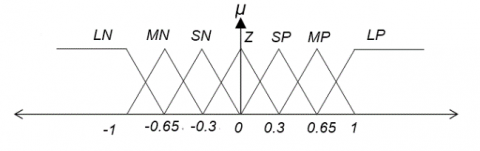Figure 7. Specification of a membership function

8.pngFigure 8. The schematic of proposed FLC of Three-level inverter

3.4 Power management

The proposed chart of the power management system is described in Figure 9, and it takes into consideration the following steps:

Step 1: define the different powers involved in our hybrid system,

Step 2: If the power supplied is greater than the requested power, in this case the excess power will be stored in the battery,

Step 3: If the requested power is greater than that generated, the following two cases are distinguish:

a) If the state of charge SOC is greater than 50 %, the storage battery devices are switched on.

b) In the opposite case, the battery stops working, which forces the delesting.

9.pngFigure 9. Flow chart of the supervisory controller

4. Simulation Results and Discussion

To study the performance of the proposed control system, we used a hysteresis to control the battery power and the FLC (Fuzzy Logic Control) to control the DC voltage of the three-level inverter (NPC) connected to the hybrid system (PV-Wind-battery). associated with an AC load.

This system is simulated using MATLAB / Simulink software under variable load conditions. The proposed system parameters are listed in Table 2, Table 3 and Table 4.

Table 2. PV and Wind Turbine Parameters

 Components Rating values Peak Power 200 W Peak Voltage 660 V Peak current 7.52 A Open Circuit Voltage 33.2 V Short Circuit Current 8.36 A Rated power (Wind) 8.5 kW Type of Generator PMSG Rated Speed 1800 Number of poles 10

Table 3. Battery and AC Load Parameters

 Components Rating values Load 1, 2, 3 (R) 7 kW, 7 kW, 3 kW Battery type Nickel Metal hydride Nominal voltage 300 V Capacity rating 6.5 AH

Table 4. DC/DC Bi-converter and Three level NPC Inverter

 Components Rating values C1=C2 2.2 mF Dc link Voltage 640 V Frequency 50 Hz Converter Inductor 5 mH DC link Voltage 640 V Converter Capacitor 2.2 mF

10.png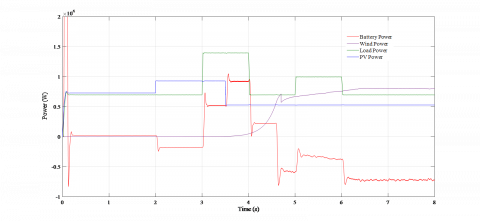Figure 10. Power generation of the hybrid system under varying AC load

11.pngFigure 11. Output voltage battery

12.png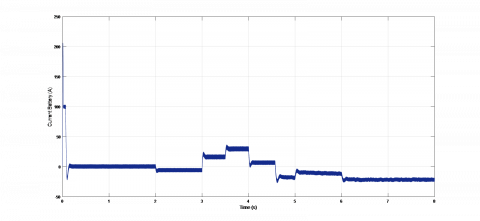Figure 12. Output current battery

13.pngFigure 13. Power battery

14.pngFigure 14. Global state of charge of battery

The proposed method is operated in some possible operating modes depending on the variable load. The system performance for this situation is shown in Figures 10 to 21.

In Figures 10, 15 and 16, the active power, current, and voltage on the load are shown. The increase of the loads can be seen clearly. First a 7 kW, then a 14 kW, then back to 7 kW, and increases to 10 kW and finally a 7 kW load were added to the system. As a result, a total load of 45 kW is supplied by the system.

15.pngFigure 15. Output current for inverter

16.pngFigure 16. Output voltage for inverter

17.pngFigure 17. Power load

18.pngFigure 18. Output power wind

19.png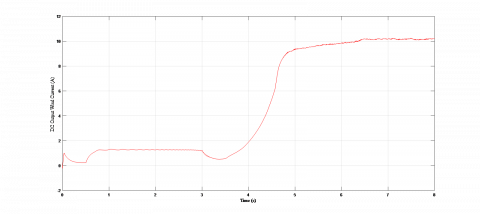Figure 19. DC output wind current

20.pngFigure 20. DC output wind voltage

21.pngFigure 21. DC bus voltage

As it can be seen in Figure 21, with changing power, the DC link voltage

is well kept constant at the specified value (640V) which constitutes an important advantage and proves the effectiveness of the proposed schema. It has allowed us to equalize the different input DC link voltages of the multilevel inverter. Then, the input voltages are practically equal by pairs as shown in Figure 21.

In Figure 14, we present the global (total) state of charge SOCG of storage devices. From 0 s to 2 s the SOC equals 60 %, and the system operates with full charge. All the storage devices are switched off because the total wind and PV generated power is higher than the load demand as shown in Figure 10.

When a SOCG decreases, and the system enters the critical mode. The supervisory controller reacts properly and switches off the load of the lowest priority in order to save the equilibrium of the overall system. If we have lack of power, we have to request for the utility of delesting process, and hence, all storage devices would be disconnected. Else, we authorize the charge mode of the storage system. These results show the efficiency of the management and the controls used for this hybrid system.

5. Conclusions

In this work a multi-source energy system for hybrid wind/solar energy and battery have been presented. Dynamic modeling and simulations of the hybrid system is proposed using SIMULINK. A hybrid energy system and its supervisory-control system was developed and tested. Load demand is met from the combination of PV array, wind turbine and the battery. A three-level inverter is used to convert output from solar and wind systems into AC power output. Circuit Breaker is used to connect and disconnet an additional load in the given time. This hybrid system is controlled to give maximum output power under all operating conditions to meet the load. Either wind or solar system is supported by the battery to meet the load. Also, simultaneous operation of wind and solar system is supported by battery for the same load.

These results show the efficiency of the management and the controls used for this hybrid system and can be implemented easily with DSP or Dspace platform.

Nomenclature
 BESS Battery Energy Storage System ed, eq direct and quadratic magneto motive force, V.. FLC Fuzzy Logic Control Ibat Battery current Id, Iq direct and quadratic stator currents, A IDC DC-link current Idc DC link current IPV Photovoltaic current L Inductive filter Lc Inductance of each stator phase, H MPPT Maximum Power Point Tracking Pbat Battery power PLoad Load power PPV Photovoltaic system output power Q Reactive power RC Resistance of each stator phase, Ω SOC State of charge of the battery Tem Electromagnetic Torque Vbat Battery voltage Vd, Vq direct and quadratic stator voltage, V Vdc DC link voltage VPV Photovoltaic system output voltage VSC Voltage Source Converters ψf Magnet flux ωr Rotor speed
References

 Aida BO, Banaei MR, Sabahi M. (2015). Hybrid PV/wind system with quinary asymmetric inverter without increasing DC-link number. Ain Shams Engineering Journal 2015: 579–592.

 Priyadarshi N. (2017). A hybrid firefly-asymmetrical fuzzy logic controller based MPPT for PV-wind-fuel grid integration. International Journal of Renewable Energy Research 7(4): 1546-1560.

 Belabbas B, Allaoui T, Tadjine M, Denai M. (2017). Power quality enhancement in hybrid photovoltaic-battery system based on three-level inverter associated with DC bus voltage control. Journal of Power Technologies 97(4): 272–282.

 Ramesh A, Kumar MS, Kumar GS, Rao VS. (2013). Analysis of Solar, wind & battery hybrid system with multilevel inverter for reduction of total harmonic distortion. International Journal of Engineering Research & Technology (IJERT) 2(11): 1595-1601.

 Ganesh P, Daivaasirvadam. M, Arundhati B. (2014). A renewable hybrid wind solar energy system fed single phase multilevel inverter. International Journal of Engineering Research & Technology (IJERT) 3(1): 1615-1621.

 Balasubramanian1 G, Singaravelu S. (2012). Fuzzy logic-based controller for a stand-alone hybrid generation system using wind and photovoltaic energy. International Journal of Advances in Engineering & Technology 3(2): 668-679.

 Das S, Akella AK. (2018). Power flow control of PV-wind-battery hybrid renewable energy systems for stand-alone application. International Journal of Renewable Energy Research 8(1): 36-43.

 Karuppa A, Samy AK, Jeyadevi S. (2014). Fuzzy logic based battery power management for PV and wind hybrid power system. Asian Journal of Science and Applied Technology 3(1): 21- 27.

 Roumila Z, Rekioua D, Rekioua T. (2017). Energy management based fuzzy logic controller of hybrid system wind/photovoltaic/diesel with storage battery. International Journal of Hydrogen Energy 42(30): 19525-19535. https://doi.org/10.1016/j.ijhydene.2017.06.006

 Chaib A, Achour D, Kesraoui M. (2016). Control of solar/wind hybrid energy system. Energy Procedia 95: 89-97. https://doi.org/10.1016/j.egypro.2016.09.028

 Jayachandran M, Ravi G. (2017). Design and optimization of hybrid micro-grid system. Energy Procedia 117: 95-103. https://doi.org/10.1016/j.egypro.2017.05.111

 Belfedhal SA, Berkouk ELM, Messlem Y. (2019). Analysis of grid connected hybrid renewable energy system. Journal of Renewable and Sustainable Energy 11: 014702. https://doi.org/10.1063/1.5054869

 Teymour HR, Sutanto D, Muttaqi KM, Ciufo P. (2014). Solar PV and battery storage integration using a new configuration of a three-level NPC inverter with advanced control strategy. IEEE Transactions on Energy Conversion 29(2): 354-365. https://doi.org/10.1109/TEC.2014.2309698

 Maouedj R, Mammeri A, Draou MD, Benyoucef B. (2015). Techno-economic analysis of standalone hybrid photovoltaic-wind system. Application in electrification of a house in Adrar region. Energy Procedia 74: 1192-1204. https://doi.org/10.1016/j.egypro.2015.07.762

 Mahesh B, Jani SM. (2014). A grid-connected hybrid generation system with multilevel inverter using SVM technique. International Journal of Computer Engineering in Research Trends 1(6): 507-512.